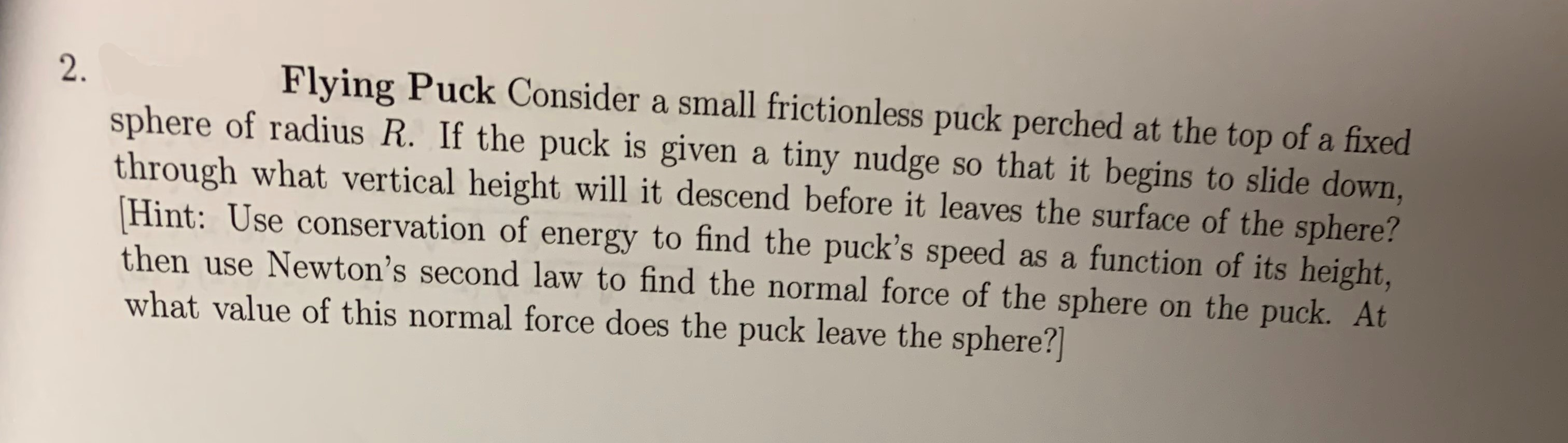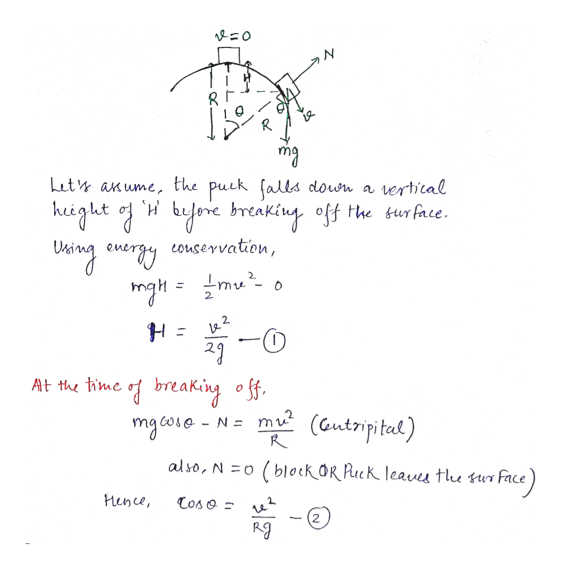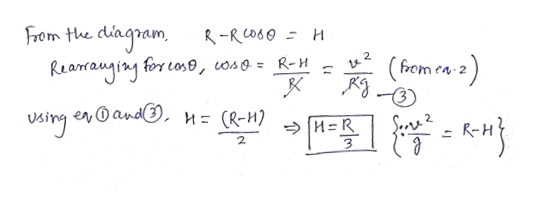Flying Puck Consider a small frictionless puck perched at the top of a fixed2.sphere of radius R. If the puck is given a tiny nudge so that it begins to slide down,through what vertical height will it descend before it leaves the surface of the sphere?Hint: Use conservation of energy to find the puck's speed as a function of its height,then use Newton's second law to find the normal force of the sphere on the puck. Atwhat value of this normal force does the puck leave the sphere?

Questionhelp_outlineImage TranscriptioncloseFlying Puck Consider a small frictionless puck perched at the top of a fixed 2. sphere of radius R. If the puck is given a tiny nudge so that it begins to slide down, through what vertical height will it descend before it leaves the surface of the sphere? Hint: Use conservation of energy to find the puck's speed as a function of its height, then use Newton's second law to find the normal force of the sphere on the puck. At what value of this normal force does the puck leave the sphere? fullscreen
Step 1

Given:

Radius of the sphere = R

Step 2

Calculating the vertical height of the puck before it breaks off the surface:help_outlineImage TranscriptioncloseN R R mg Lut anume, the puck (alls down a heeghtf a cnergy couservation, ertical bjene breaking off the turface mgt=mu 2. 2 At the th of breaki off. i'me N= mu(autripital) mg coe R also, N o (blockOR Puck leavų tlu tur face Hence Cos Z fullscreen
Step 3

Calculating the final va...help_outlineImage TranscriptioncloseFrom the . diagoam Reanrauginy for cos0, wso = R-H R-RCO60 H 2 from ea 2 1 0sing enandO, n= (R-M) R Srs 2. 3 fullscreen

Want to see the full answer?

See Solution

Want to see this answer and more?

Our solutions are written by experts, many with advanced degrees, and available 24/7

See Solution
Tagged in

Newtons Laws of Motion